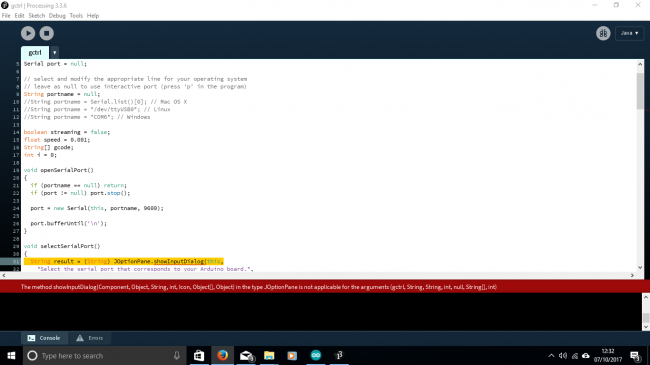#### Howdy, Stranger!

We are about to switch to a new forum software. Until then we have removed the registration on this forum.

# Why can't I get the option pane to show.

edited October 2017 in Arduino

A newby and my first time using Processing and I can't see how I can resolve this. I'm trying to set up a CNC drawing setup in arduino uno and the Processing option pane is not displaying correctly. I've attached a screenshot and it appears a fault is shown at line 31. Would greatly appreciate any helpTagged:

• We cannot read your code. This is why screenshots are not good. Instead, please copy and paste your code here in the forum, making sure you format your code properly (Read the sticky posts). Also and copy the error that is shown in the console in your post below your code.

Kf

• Thanks for your speedy reply and forgive my ignorance. I hope you can make something of this:

``````import java.awt.event.KeyEvent;
import javax.swing.JOptionPane;
import processing.serial.*;

Serial port = null;

// select and modify the appropriate line for your operating system
// leave as null to use interactive port (press 'p' in the program)
String portname = null;
//String portname = Serial.list(); // Mac OS X
//String portname = "/dev/ttyUSB0"; // Linux
//String portname = "COM6"; // Windows

boolean streaming = false;
float speed = 0.001;
String[] gcode;
int i = 0;

void openSerialPort()
{
if (portname == null) return;
if (port != null) port.stop();

port = new Serial(this, portname, 9600);

port.bufferUntil('\n');
}

void selectSerialPort()
{
String result = (String) JOptionPane.showInputDialog(this,
"Select the serial port that corresponds to your Arduino board.",
"Select serial port",
JOptionPane.PLAIN_MESSAGE,
null,
Serial.list(),
0);

if (result != null) {
portname = result;
openSerialPort();
}
}

void setup()
{
size(500, 250);
openSerialPort();
}

void draw()
{
background(0);
fill(255);
int y = 24, dy = 12;
text("INSTRUCTIONS", 12, y); y += dy;
text("p: select serial port", 12, y); y += dy;
text("1: set speed to 0.001 inches (1 mil) per jog", 12, y); y += dy;
text("2: set speed to 0.010 inches (10 mil) per jog", 12, y); y += dy;
text("3: set speed to 0.100 inches (100 mil) per jog", 12, y); y += dy;
text("arrow keys: jog in x-y plane", 12, y); y += dy;
text("page up & page down: jog in z axis", 12, y); y += dy;
text("\$: display grbl settings", 12, y); y+= dy;
text("h: go home", 12, y); y += dy;
text("0: zero machine (set home to the current location)", 12, y); y += dy;
text("g: stream a g-code file", 12, y); y += dy;
text("x: stop streaming g-code (this is NOT immediate)", 12, y); y += dy;
y = height - dy;
text("current jog speed: " + speed + " inches per step", 12, y); y -= dy;
text("current serial port: " + portname, 12, y); y -= dy;
}

void keyPressed()
{
if (key == '1') speed = 0.001;
if (key == '2') speed = 0.01;
if (key == '3') speed = 0.1;

if (!streaming) {
if (keyCode == LEFT) port.write("G91\nG20\nG00 X-" + speed + " Y0.000 Z0.000\n");
if (keyCode == RIGHT) port.write("G91\nG20\nG00 X" + speed + " Y0.000 Z0.000\n");
if (keyCode == UP) port.write("G91\nG20\nG00 X0.000 Y" + speed + " Z0.000\n");
if (keyCode == DOWN) port.write("G91\nG20\nG00 X0.000 Y-" + speed + " Z0.000\n");
if (keyCode == KeyEvent.VK_PAGE_UP) port.write("G91\nG20\nG00 X0.000 Y0.000 Z" + speed + "\n");
if (keyCode == KeyEvent.VK_PAGE_DOWN) port.write("G91\nG20\nG00 X0.000 Y0.000 Z-" + speed + "\n");
if (key == 'h') port.write("G90\nG20\nG00 X0.000 Y0.000 Z0.000\n");
if (key == 'v') port.write("\$0=75\n\$1=74\n\$2=75\n");
//if (key == 'v') port.write("\$0=100\n\$1=74\n\$2=75\n");
if (key == 's') port.write("\$3=10\n");
if (key == 'e') port.write("\$16=1\n");
if (key == 'd') port.write("\$16=0\n");
if (key == '0') openSerialPort();
if (key == 'p') selectSerialPort();
if (key == '\$') port.write("\$\$\n");
}

if (!streaming && key == 'g') {
gcode = null; i = 0;
File file = null;
selectInput("Select a file to process:", "fileSelected", file);
}

if (key == 'x') streaming = false;
}

void fileSelected(File selection) {
if (selection == null) {
println("Window was closed or the user hit cancel.");
} else {
println("User selected " + selection.getAbsolutePath());
if (gcode == null) return;
streaming = true;
stream();
}
}

void stream()
{
if (!streaming) return;

while (true) {
if (i == gcode.length) {
streaming = false;
return;
}

if (gcode[i].trim().length() == 0) i++;
else break;
}

println(gcode[i]);
port.write(gcode[i] + '\n');
i++;
}

void serialEvent(Serial p)
{
println(s.trim());

if (s.trim().startsWith("ok")) stream();
if (s.trim().startsWith("error")) stream(); // XXX: really?
}
``````

I'm not sure how to copy the error in the console but it's:

The Function "showInputDialog()" expects parameters like: "showInputDialog(Components, Objects, String, int, Icon, Object[], Object )"

Thanks Again

• Try this change in line 31:

`String result = (String) JOptionPane.showInputDialog(frame`,

Also I will review the design of your code as line 21 might be unnecessary and potentially problematic.

Kf